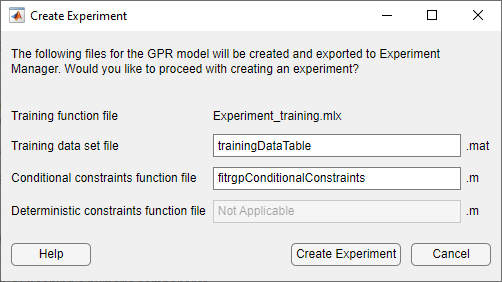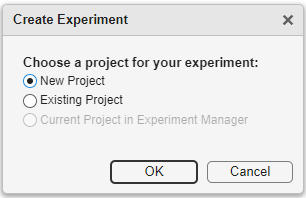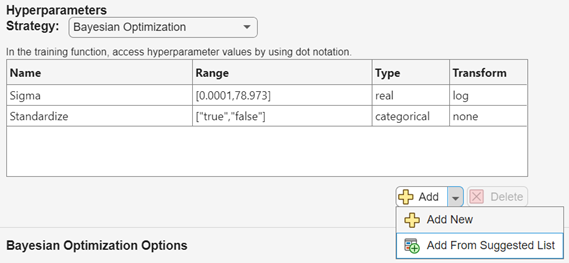## Export Model from Regression Learner to Experiment Manager

After training a regression model in Regression Learner, you can export the model to Experiment Manager to perform multiple experiments. By default, Experiment Manager uses Bayesian optimization to tune the model in a process similar to training optimizable models in Regression Learner. (For more information, see Hyperparameter Optimization in Regression Learner App.) Consider exporting a model to Experiment Manager when you want to do any of the following:

• Adjust hyperparameter search ranges during hyperparameter tuning.

• Change the training data.

• Adjust the preprocessing steps that precede model fitting.

• Tune hyperparameters using a different metric.

For a workflow example, see Tune Regression Model Using Experiment Manager.

Note that if you have a Statistics and Machine Learning Toolbox™ license, you do not need a Deep Learning Toolbox™ license to use the Experiment Manager app.

### Export Regression Model

To create an Experiment Manager experiment from a model trained in Regression Learner, select the model in the Models pane. On the Learn tab, in the Export section, click and select Create Experiment.

Note

This option is not supported for linear regression models.

In the Create Experiment dialog box, modify the filenames or accept the default values.The app exports the following files to Experiment Manager:

• Training function — This function trains a regression model using the model hyperparameters specified in the Experiment Manager app and records the resulting metrics and visualizations. For each trial of the experiment, the app calls the training function with a new combination of hyperparameter values, selected from the hyperparameter search ranges specified in the app. The app saves the returned trained model, which you can export to the MATLAB® workspace after training is complete.

• Training data set — This `.mat` file contains the full data set used in Regression Learner (including training and validation data, but excluding test data). Depending on how you imported the data into Regression Learner, the data set is contained in either a table named `dataTable` or two separate variables named `predictorMatrix` and `responseData`.

• Conditional constraints function — For some models, a conditional constraints function is required to tune model hyperparameters using Bayesian optimization. Conditional constraints enforce one of these conditions:

• When some hyperparameters have certain values, other hyperparameters are set to given values.

• When some hyperparameters have certain values, other hyperparameters are set to `NaN` values (for numeric hyperparameters) or `<undefined>` values (for categorical hyperparameters).

• Deterministic constraints function — For some models, a deterministic constraints function is required to tune model hyperparameters using Bayesian optimization. A deterministic constraints function returns a `true` value when a point in the hyperparameter search space is feasible (that is, the problem is valid or well defined at this point) and a `false` value otherwise. For more information, see Deterministic Constraints — XConstraintFcn.

After you click , the app opens Experiment Manager. The Experiment Manager app then opens a dialog box in which you can choose to use a new or existing project for your experiment.### Select Hyperparameters

In Experiment Manager, use different hyperparameters and hyperparameter search ranges to tune your model. On the tab for your experiment, in the Hyperparameters section, click to add a hyperparameter to the model tuning process. In the table, double-click an entry to adjust its value.When you use the default Bayesian optimization strategy for model tuning, specify these properties of the hyperparameters used in the experiment:

• Name — Enter a valid hyperparameter name.

• Range — For a real- or integer-valued hyperparameter, enter a two-element vector that gives the lower bound and upper bound of the hyperparameter. For a categorical hyperparameter, enter an array of strings or a cell array of character vectors that lists the possible values of the hyperparameter.

• Type — Select `real` for a real-valued hyperparameter, `integer` for an integer-valued hyperparameter, or `categorical` for a categorical hyperparameter.

• Transform — Select `none` to use no transform or `log` to use a logarithmic transform. When you select `log`, the hyperparameter values must be positive. With this setting, the Bayesian optimization algorithm models the hyperparameter on a logarithmic scale.

The following table provides information on the hyperparameters you can tune in the app for each model type.

Model TypeFitting FunctionHyperparameters
Tree`fitrtree`

For more information, see `OptimizeHyperparameters`.

Gaussian Process Regression`fitrgp`

For more information, see `OptimizeHyperparameters`.

SVM`fitrsvm`

For more information, see `OptimizeHyperparameters`.

Efficient Linear`fitrlinear`

For more information, see `OptimizeHyperparameters`.

Kernel`fitrkernel`

For more information, see `OptimizeHyperparameters`.

Ensemble`fitrensemble`

For more information, see `OptimizeHyperparameters`.

Neural Network`fitrnet`

For more information, see `OptimizeHyperparameters`.

In the MATLAB Command Window, you can use the `hyperparameters` function to get more information about the hyperparameters available for your model and their default search ranges. Specify the fitting function, the training predictor data, and the training response variable in the call to the `hyperparameters` function.

### (Optional) Customize Experiment

In your experiment, you can change more than the model hyperparameters. In most cases, experiment customization requires editing the training function file before running the experiment. For example, to change the training data set, preprocessing steps, returned metrics, or generated visualizations, you must update the training function file. To edit the training function, click in the Training Function section on the experiment tab. For an example that includes experiment customization, see Tune Regression Model Using Experiment Manager.

Some experiment customization steps do not require editing the training function file. For example, you can change the strategy for model tuning, adjust the Bayesian optimization options, or change the metric used to perform Bayesian optimization.

#### Change Strategy for Model Tuning

Instead of using Bayesian optimization to search for the best hyperparameter values, you can sweep through a range of hyperparameter values. On the tab for your experiment, in the Hyperparameters section, set Strategy to ```Exhaustive Sweep```. In the hyperparameter table, enter the names and values of the hyperparameters to use in the experiment. Hyperparameter values must be scalars or vectors with numeric, logical, or string values, or cell arrays of character vectors. For example, these are valid hyperparameter specifications, depending on your model:

• `0.01`

• `0.01:0.01:0.05`

• `[0.01 0.02 0.04 0.08]`

• `["Bag","LSBoost"]`

• `{'gaussian','linear','polynomial'}`

When you run the experiment, Experiment Manager trains a model using every combination of the hyperparameter values specified in the table.

When you use Bayesian optimization, you can specify the duration of your experiment. On the tab for your experiment, in the Hyperparameters section, ensure that Strategy is set to ```Bayesian Optimization```. In the Bayesian Optimization Options section, enter the maximum time in seconds and the maximum number of trials to run. Note that the actual run time and number of trials in your experiment can exceed these settings because Experiment Manager checks these options only when a trial finishes executing.

You can also specify the acquisition function for the Bayesian optimization algorithm. In the Bayesian Optimization Options section, click Advanced Options. Select an acquisition function from the Acquisition Function Name list. The default value for this option is `expected-improvement-plus`. For more information, see Acquisition Function Types.

Note that if you edit the training function file so that a new deterministic constraints function or conditional constraints function is required, you can specify the new function names in the Advanced Options section.

#### Change Metric Used to Perform Bayesian Optimization

By default, the app uses Bayesian optimization to try to find the combination of hyperparameter values that minimizes the validation RMSE. You can specify to maximize the validation R-squared value instead. On the tab for your experiment, in the Metrics section, specify to optimize the `ValidationRSquared` value. Set the Direction to `Maximize`.

Note that if you edit the training function file to return another metric, you can specify it in the Metrics section. Ensure that the Direction is appropriate for the given metric.

### Run Experiment

When are you ready to run your experiment, you can run it either sequentially or in parallel.

• If you have Parallel Computing Toolbox™, Experiment Manager can perform computations in parallel. On the Experiment Manager tab, in the Execution section, select `Simultaneous` from the Mode list.

Note

Parallel computations with a thread pool are not supported in Experiment Manager.

• Otherwise, use the default Mode option of `Sequential`.

On the Experiment Manager tab, in the Run section, click .